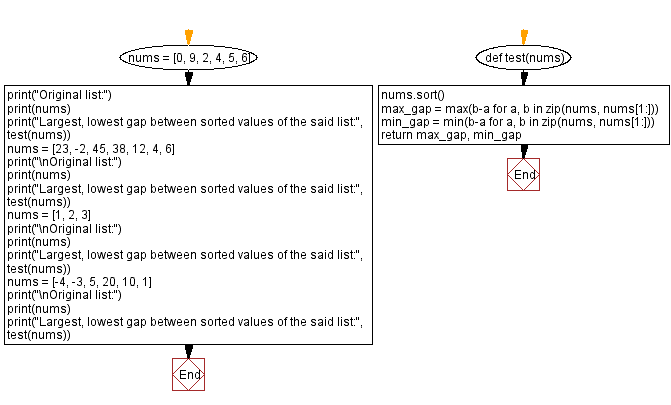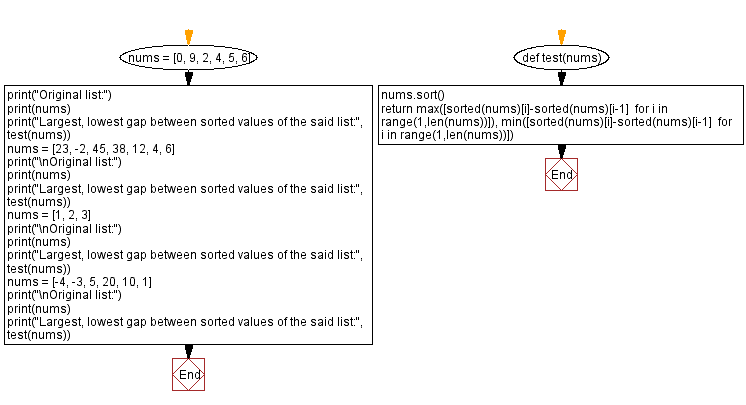﻿ Python - Largest, lowest gap between sorted values of a list# Python Exercises: Largest, lowest gap between sorted values of a list

## Python List: Exercise - 277 with Solution

Write a Python program to calculate the largest and gap lowest between sorted elements of a list of integers.

Sample Data:
{1, 2 ,9, 0, 4, 6} -> 3
{23, -2, 45, 38, 12, 4, 6} -> 15

Sample Solution-1:

Python Code:

``````def test(nums):
nums.sort()
max_gap = max(b-a for a, b in zip(nums, nums[1:]))
min_gap = min(b-a for a, b in zip(nums, nums[1:]))
return max_gap, min_gap
nums = [0, 9, 2, 4, 5, 6]
print("Original list:")
print(nums)
print("Largest, lowest gap between sorted values of the said list:", test(nums))
nums = [23, -2, 45, 38, 12, 4, 6]
print("\nOriginal list:")
print(nums)
print("Largest, lowest gap between sorted values of the said list:", test(nums))
nums = [1, 2, 3]
print("\nOriginal list:")
print(nums)
print("Largest, lowest gap between sorted values of the said list:", test(nums))
nums = [-4, -3, 5, 20, 10, 1]
print("\nOriginal list:")
print(nums)
print("Largest, lowest gap between sorted values of the said list:", test(nums))
```
```

Sample Output:

```Original list:
[0, 9, 2, 4, 5, 6]
Largest, lowest gap between sorted values of the said list: (3, 1)

Original list:
[23, -2, 45, 38, 12, 4, 6]
Largest, lowest gap between sorted values of the said list: (15, 2)

Original list:
[1, 2, 3]
Largest, lowest gap between sorted values of the said list: (1, 1)

Original list:
[-4, -3, 5, 20, 10, 1]
Largest, lowest gap between sorted values of the said list: (10, 1)
```

Flowchart:## Visualize Python code execution:

The following tool visualize what the computer is doing step-by-step as it executes the said program:

Sample Solution-2:

Python Code:

``````def test(nums):
nums.sort()
return max([sorted(nums)[i]-sorted(nums)[i-1]  for i in range(1,len(nums))]), min([sorted(nums)[i]-sorted(nums)[i-1]  for i in range(1,len(nums))])
nums = [0, 9, 2, 4, 5, 6]
print("Original list:")
print(nums)
print("Largest, lowest gap between sorted values of the said list:", test(nums))
nums = [23, -2, 45, 38, 12, 4, 6]
print("\nOriginal list:")
print(nums)
print("Largest, lowest gap between sorted values of the said list:", test(nums))
nums = [1, 2, 3]
print("\nOriginal list:")
print(nums)
print("Largest, lowest gap between sorted values of the said list:", test(nums))
nums = [-4, -3, 5, 20, 10, 1]
print("\nOriginal list:")
print(nums)
print("Largest, lowest gap between sorted values of the said list:", test(nums))
```
```

Sample Output:

```Original list:
[0, 9, 2, 4, 5, 6]
Largest, lowest gap between sorted values of the said list: (3, 1)

Original list:
[23, -2, 45, 38, 12, 4, 6]
Largest, lowest gap between sorted values of the said list: (15, 2)

Original list:
[1, 2, 3]
Largest, lowest gap between sorted values of the said list: (1, 1)

Original list:
[-4, -3, 5, 20, 10, 1]
Largest, lowest gap between sorted values of the said list: (10, 1)
```

Flowchart:## Visualize Python code execution:

The following tool visualize what the computer is doing step-by-step as it executes the said program:

Python Code Editor:

Have another way to solve this solution? Contribute your code (and comments) through Disqus.

Previous Python Exercise: Find the largest odd number in a list of integers.
Next Python Exercise: Sum of missing numbers of a list of integers.

What is the difficulty level of this exercise?

Test your Programming skills with w3resource's quiz.

﻿

## Python: Tips of the Day

Getting sorted iterable (can sort by "compare" function):

```>>> a = [1, 2, -3]
>>> sorted(a)
[-3, 1, 2]

>>> sorted(a,key=abs)
[1, 2, -3]
```